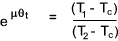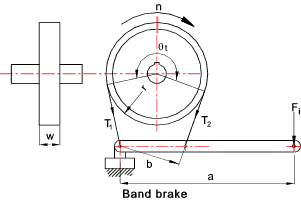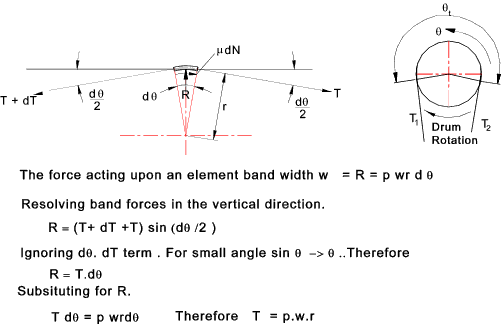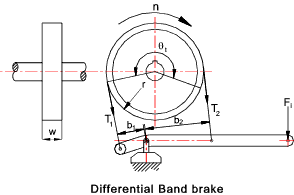Brakes

Band Brakes

Introduction

This is a very simple type of brake using the principle that a band is wrapped part round a rotating drum.  Tension can be applied to the band using a lever.    The restraining torque results from the difference in tension between the two ends of the belt.

The two band brake options are shown below

Nomenclature
 F = Applied Force (N) P = Brake Power kW M = Torque (Nm) F i = Actuating Force (N) μ = Coefficient of Friction. θt = Total band lap angle (rad) w = Band width a = Pivot- Actuating force radius (m) b = Distance from tensioning belt to fulcrum point n = Rotational Speed (RPM) T1 = Maximum band tension(N) T1 = Minimum band tension(N) Tc = Band tension associated with centrifugal force (N) p = pressure between band and drum surface (N/m2) pmax = maximum pressure for friction surface(N/m2)

Band Brake

The principles of operation of the band brake is the same as for belt drives.. refer to information on page Flat Belt Drives
The formula below relating the band tensions (T1, T2 ) the lap angle θ t and the coefficient of friction μ is derived on the page on Belt drives).The tension due to the belt rotational inertia for Tc = 0 because the band is not moving for the brake application. The relevant formula is therefore

T1 /T2 = e μθ tFor the Normal Band Brake arrangement shown the relationship between the actuating force and the slack side belt tension is

Fi = T2 . b /a

Brake Torque Capacity

The torque capacity of the band brake =

M = (T1 - T2 ) r

Maximum Belt TensionThe maximum tension in a belt is provided by the formula below.

T1_max = pmax.w.r

Differential Band Brake

The differential band brake as shown below can be configured to be self energising and can be arranged to operate in either direction..The actuating force equation obtained by summing moments about the pivot points is shown below

Fi = ( b 2 T 2 - b 1 T 1 ) / a

If b 2 T 2 = b 1 T 1 the zero actuating force is required and the brake is self locking.  It is also apparent that the brake is effectively free wheeling in the opposite direction.  The differential brake can therefore be arranged to enable rotation in one direction only.

 Links to Brake Design Clutches and Brakes ...A detailed selection and design guide download book Mayr ...Brakes and Clutches RingSpann .Disc Brakes Warner ..Brake /Clutch Information/Data sheets Twiflex ..Brake /Clutch Information/Data sheets- Registration Required Friction Technology ..Information/Data sheets- Registration Required pintschbubenzer-Drum_Band brakes .. Downloads of various band brake systems- specifications

Brakes